# Gaseous Introduction (Part - 1) Chemistry Notes | EduRev

## Chemistry : Gaseous Introduction (Part - 1) Chemistry Notes | EduRev

The document Gaseous Introduction (Part - 1) Chemistry Notes | EduRev is a part of the Chemistry Course Physical Chemistry.
All you need of Chemistry at this link: Chemistry

1. GASEOUS STATE

Gas is one of the four fundamental states of matter (the others being solid, liquid, and plasma). A pure gas may be made up of individual atoms (e.g. a noble gas like neon), elemental molecules made from one type of atom (e.g. oxygen), or compound molecules made from a variety of atoms (e.g. carbon dioxide).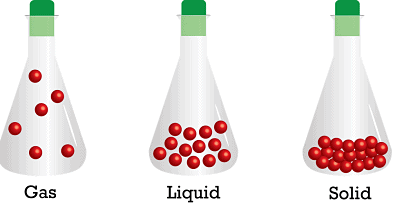Arrangement of atoms in different states

A gas mixture would contain a variety of pure gases much like the air. What distinguishes a gas from liquids and solids is the vast separation of the individual gas particles? This separation usually makes a colorless gas invisible to the human observer.

The interaction of gas particles in the presence of electric and gravitational fields are considered negligible as indicated by the constant velocity vectors in the image.

The gaseous state of matter is found between the liquid and plasma states, the latter of which provides the upper-temperature boundary for gases. Bounding lower end of the temperature scale lies degenerative quantum gases which are gaining increasing attention. High-density atomic gases super cooled to incredibly low temperatures are classified by their statistical behavior as either a Bose gas or a Fermi gas. For a comprehensive listing of these exotic states of matter see the list of states of matter.

The state is characterized by sensitivity of volume change with the change of pressure and temperature. It is due to the large distance between molecules as compared to their own dimensions. There exist weak Vander Waal's forces, molecules move independently of each other with speed about 400 ms–1.

2. Physical Characteristics

Because most gases are difficult to observe directly, they are described through the use of four physical properties or macroscopic characteristics: pressure, volume, number of particles (chemists group them by moles) and temperature.

These four characteristics were repeatedly observed by scientists such as Robert Boyle, Jacques Charles, John Dalton, Joseph Gay-Lussac and Amedeo Avogadro for a variety of gases in various settings. Their detailed studies ultimately led to a mathematical relationship among these properties expressed by the ideal gas law (see simplified models section below).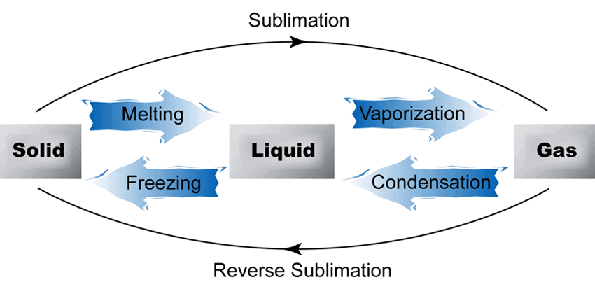Interconversion between states0

Gas particles are widely separated from one another, and consequently, have weaker inter-molecular bonds than liquids or solids. These inter-molecular forces result from electrostatic interactions between gas particles. Like-charged areas of different gas particles repel, while oppositely charged regions of different gas particles attract one another; gases that contain permanently charged ions are known as plasmas.

Gaseous compounds with polar covalent bonds contain permanent charge imbalances and so experience relatively strong inter-molecular forces, although the molecule while the compound's net charge remains neutral.

Transient, randomly induced charges exist across non-polar covalent bonds of molecules and electrostatic interactions caused by them are referred to as Van der Waals forces. The interaction of these inter-molecular forces varies within a substance which determines many of the physical properties unique to each gas.

A comparison of boiling points for compounds formed by ionic and covalent bonds leads us to this conclusion. Drifting smoke particles in the image provides some insight into low-pressure gas behavior.

Compared to the other states of matter, gases have low density and viscosity. Pressure and temperature influence the particles within a certain volume. This variation in particle separation and speed is referred to as compressibility.

This particle separation and size influences optical properties of gases as can be found in the following list of refractive indices. Finally, gas particles spread apart or diffuse in order to homogeneously distribute themselves throughout any container.

3. The parameter associated with the gas

A. Pressure- The symbol used to represent pressure in equations is "p" or "P" with SI units of pascals.

When describing a container of gas, the term pressure (or absolute pressure) refers to the average force per unit area that the gas exerts on the surface of the container. Within this volume, it is sometimes easier to visualize the gas particles moving in straight lines until they collide with the container (see diagram at top of the article).

The force imparted by a gas particle into the container during this collision is the change in momentum of the particle. During the collision, only normal component of velocity changes. A particle traveling parallel to the wall does not change its momentum. Therefore, the average force on a surface must be the average change in linear momentum from all of these gas particle collisions.

Pressure is the sum of all the normal components of force exerted by the particles impacting the walls of the container divided by the surface area of the wall.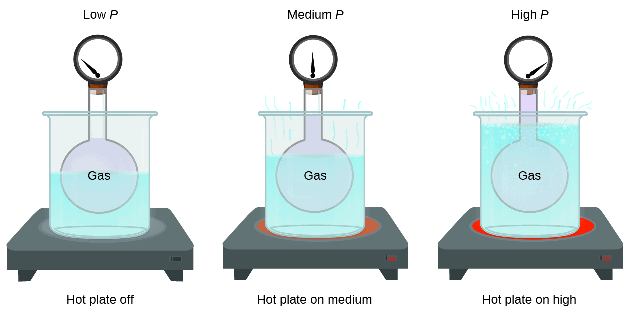Pressure and temperature relation

B. Temperature- The symbol used to represent temperature in equations is T with SI units of kelvins.

The speed of a gas particle is proportional to its absolute temperature. The volume of the balloon in the video shrinks when the trapped gas particles slow down with the addition of extremely cold nitrogen. The temperature of any physical system is related to the motions of the particles (molecules and atoms) which make up the [gas] system.

In statistical mechanics, temperature is the measure of the average kinetic energy stored in a particle. The methods of storing this energy are dictated by the degrees of freedom of the particle itself (energy modes). Kinetic energy added (endothermic process) to gas particles by way of collisions produces linear, rotational, and vibrational motion.

In contrast, a molecule in a solid can only increase its vibrational modes with the addition of heat as the lattice crystal structure prevents both linear and rotational motions. These heated gas molecules have a greater speed range which constantly varies due to constant collisions with other particles.

The speed range can be described by the Maxwell–Boltzmann distribution. Use of this distribution implies ideal gases near thermodynamic equilibrium for the system of particles being considered.

C. Specific Volume- The symbol used to represent specific volume in equations is "v" with SI unit s of cubic meters per kilogram.

The symbol used to represent volume in equations is "V" with SI units of cubic meters.

When performing a thermodynamic analysis, it is typical to speak of intensive and extensive properties. Properties which depend on the amount of gas (either by mass or volume) are called extensive properties, while properties that do not depend on the amount of gas are called intensive properties.

Specific volume is an example of an intensive property because it is the ratio of volume occupied by a unit of mass of a gas that is identical throughout a system at equilibrium. 1000 atoms a gas occupy the same space as any other 1000 atoms for any given temperature and pressure. This concept is easier to visualize for solids such as iron which is incompressible compared to gases. Since a gas fills any container in which it is placed, volume is an extensive property.

D. Density- The symbol used to represent density in equations is ρ (rho) with SI units of kilograms per cubic meter. This term is the reciprocal of specific volume.

Since gas molecules can move freely within a container, their mass is normally characterized by density.

Density is the amount of mass per unit volume of a substance or the inverse of specific volume. For gases, the density can vary over a wide range because the particles are free to move closer together when constrained by pressure or volume.

This variation of density is referred to as compressibility. As pressure and temperature, density is a state variable of a gas and the change in density during any process is governed by the laws of thermodynamics.

For a static gas, the density is the same throughout the entire container. Density is, therefore, a scalar quantity. It can be shown by the kinetic theory that the density is inversely proportional to the size of the container in which a fixed mass of gas is confined. In this case of a fixed mass, density decreases as the volume increases.

4. Microscopic View

If one could observe a gas under a powerful microscope, one would see a collection of particles (molecules, atoms, ions, electrons, etc.) without any definite shape or volume that are in more or less random motion. These neutral gas particles only change direction when they collide with another particle or with the sides of the container.

In an ideal gas, these collisions are perfectly elastic. This particle or microscopic view of a gas is described by the Kinetic-molecular theory. The assumptions behind this theory can be found in the postulates section of Kinetic Theory.

5. Kinetic Gas Theory

Kinetic theory provides insight into the macroscopic properties of gases by considering their molecular composition and motion. Starting with the definitions of momentum and kinetic energy, one can use the conservation of momentum and geometric relationships of a cube to relate macroscopic system properties of temperature and pressure to the microscopic property of kinetic energy per molecule. The theory provides averaged values for these two properties.

The theory also explains how the gas system responds to change. For example, as a gas is heated from absolute zero, when it is (in theory) perfectly still, its internal energy (temperature) is increased.

As a gas is heated, the particles speed up and its temperature rises. This results in greater numbers of collisions with the container per unit time due to the higher particle speeds associated with elevated temperatures. The pressure increases in proportion to the number of collisions per unit time.

1. Postulates of an ideal gas:

The essential postulates are :

• A gas consists of a large number of very small spherical tiny particles, which may be identified with the molecules.
• The molecules of a given gas are completely identical in size, shape, and mass.
• The volume occupied by the molecules is negligible in comparison to the total volume of the gas.
• The molecules are in rapid motion which is completely random. During their motion, they collide with one another and with the sides of the vessel. The pressure of the gas is due to the collisions of molecules with the sides of the vessel.
• The molecules are perfectly elastic, i.e. there occurs no loss of energy when they collide with one another and with the sides of the vessel.
• The laws of classical mechanics, in particular, Newton’s second law of motion, are applicable to the molecules in motion.
• There is no force of attraction or repulsion among the molecules, i.e. they are moving independently of one another.
• At any instant, a given molecule can have energy ranging from a small value to a very large value, but the average kinetic energy remains constant for a given temperature.  i.e. the average kinetic energy is proportional to the absolute temperature of the gas.

2. A derivative of the Kinetic Gas Equation

Imagine a cube of edge-length l, containing N molecule, each having a mass of m.

The velocity u1 of any molecule may be resolved into three-component velocities designated as ux, uy and uz.

The component velocities are related by the expression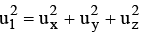…………….(1)

Considering the x-component motion of the molecule, we will have

Momentum of molecule before collision with the side ABCD = mux.

Momentum of the molecule after collision with the side ABCD = –mux.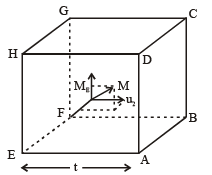Change of momentum of the molecule in a single collision with the side ABCD = |2mux|.

The total change of momentum per unit time due to such impacts is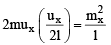The area of the wall is l2.  Hence the pressure exerted due to the collision of the x-component velocity of a single molecule with the side ABCD is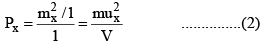Where V is the volume of the vessel.

Since each molecule will exert similar pressure, the total pressure exerted on the wall ABCD will be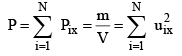...............(3)

Defining the mean square speed as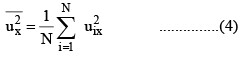we can write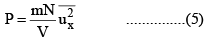Since the directions x, y and z are equivalent will also have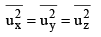But from eq. (1) we have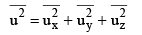...(7)

From eq. (6) and (7) we have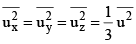...(8)

Substituting this in eq. (5) we get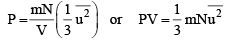....(9)

Offer running on EduRev: Apply code STAYHOME200 to get INR 200 off on our premium plan EduRev Infinity!

## Physical Chemistry

77 videos|83 docs|32 tests

,

,

,

,

,

,

,

,

,

,

,

,

,

,

,

,

,

,

,

,

,

;